﻿ 电网用户服务满意度提升策略研究 Research on the Improvement Strategy of Grid User Service Satisfaction

Vol. 08  No. 02 ( 2019 ), Article ID: 28876 , 8 pages
10.12677/AAM.2019.82028

Research on the Improvement Strategy of Grid User Service Satisfaction

Yao Zhou, Lihong Yang, Yanran Lin

School of Mathematics, South China University of Technology, Guangzhou Guangdong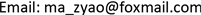Received: Jan. 24th, 2019; accepted: Feb. 8th, 2019; published: Feb. 15th, 2019ABSTRACT

Based on the data of customer satisfaction survey in a city, this paper establishes evaluation indicators of grid user satisfaction such as power outage processing and power supply security, and constructs a user satisfaction analysis model to quantify the user experience satisfaction of the city's power supply bureau. Based on the solution results, the correlation between indicators is discussed, and the satisfaction level and cause analysis are carried out. Differentiated guidance and suggestions for customer service improvement and targeted personalized service are provided to improve grid user satisfaction.

Keywords:User Satisfaction, Satisfaction Analysis Model, Cause Analysis, Personalized Service1. 引言

2. 结构方程模型理论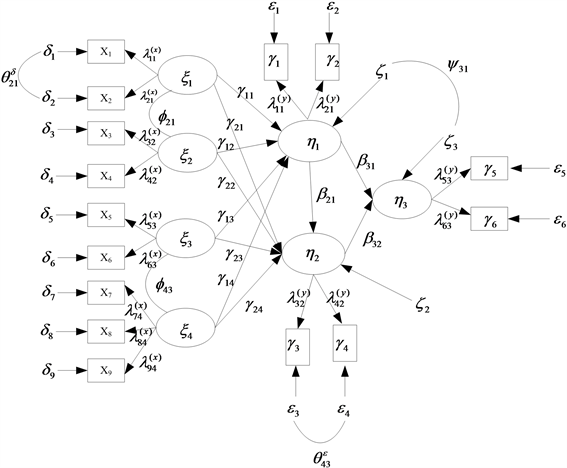Figure 1. LISREL causal model path map

$\left\{\begin{array}{l}{X}_{1}={\lambda }_{11}{\xi }_{1}+{\delta }_{1}\\ {X}_{2}={\lambda }_{21}{\xi }_{1}+{\delta }_{2}\\ {X}_{3}={\lambda }_{31}{\xi }_{1}+{\delta }_{3}\\ {X}_{4}={\lambda }_{42}{\xi }_{2}+{\delta }_{4}\\ {X}_{5}={\lambda }_{53}{\xi }_{3}+{\delta }_{5}\\ {X}_{6}={\lambda }_{63}{\xi }_{3}+{\delta }_{6}\\ {X}_{7}={\lambda }_{74}{\xi }_{4}+{\delta }_{7}\\ {X}_{8}={\lambda }_{84}{\xi }_{4}+{\delta }_{8}\\ {X}_{9}={\lambda }_{94}{\xi }_{4}+{\delta }_{9}\end{array}$ (1)

$\left\{\begin{array}{l}{Y}_{1}={\lambda }_{11}{\eta }_{1}+{\epsilon }_{1}\\ {Y}_{2}={\lambda }_{21}{\eta }_{1}+{\epsilon }_{2}\\ {Y}_{3}={\lambda }_{32}{\eta }_{2}+{\epsilon }_{3}\\ {Y}_{4}={\lambda }_{42}{\eta }_{2}+{\epsilon }_{4}\\ {Y}_{5}={\lambda }_{53}{\eta }_{3}+{\epsilon }_{5}\\ {Y}_{6}={\lambda }_{63}{\eta }_{3}+{\epsilon }_{6}\end{array}$ (2)

$\left\{\begin{array}{l}{\eta }_{1}={\gamma }_{11}{\xi }_{1}+{\gamma }_{12}{\xi }_{2}+{\gamma }_{13}{\xi }_{3}+{\gamma }_{14}{\xi }_{4}+\zeta \\ {\eta }_{2}={\gamma }_{21}{\xi }_{1}+{\gamma }_{22}{\xi }_{2}+{\gamma }_{23}{\xi }_{3}+{\gamma }_{24}{\xi }_{4}+{\beta }_{21}{\eta }_{1}+{\zeta }_{2}\\ {\eta }_{3}={\beta }_{31}{\eta }_{1}+{\beta }_{32}{\eta }_{2}+{\zeta }_{3}\end{array}$ (3)

3. 模型构建

4. 模型求解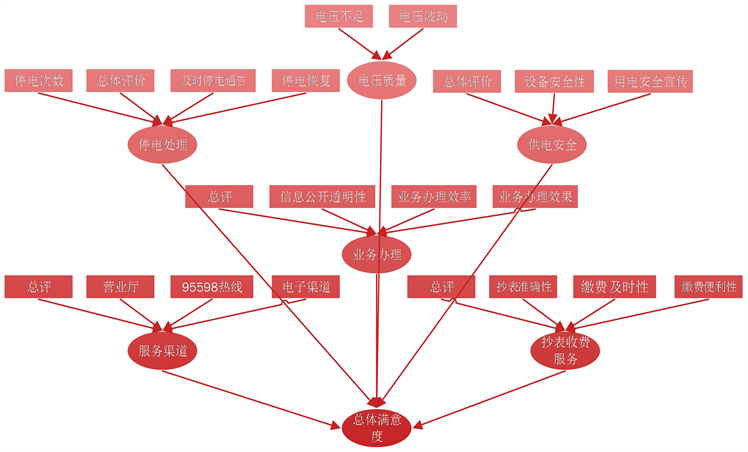Figure 2. Schematic diagram of structural equation model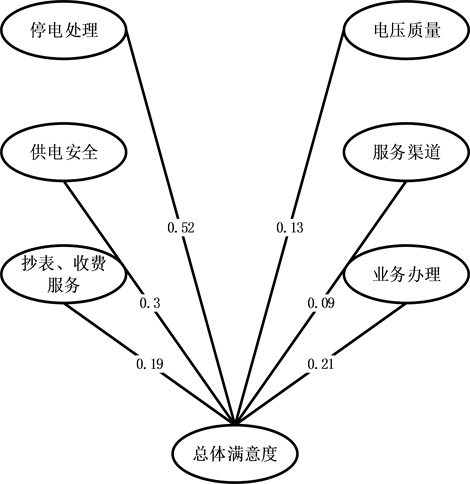Figure 3. Model weight mapTable 1. Summary of indicator weights at all levels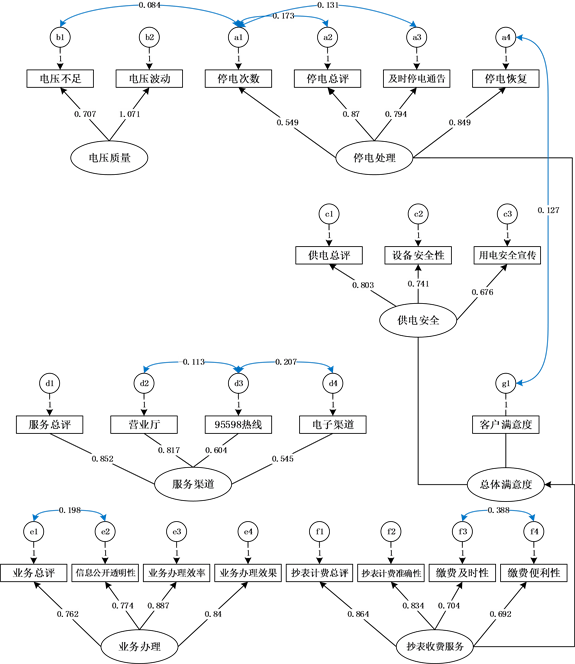Figure 4. Model weight mapTable 3. Summary of indicator weights at all levels

CMIN/DF < 2，CF I> 0.9，且与1相当接近，RMSEA < 0.05说明模型非常好。

5. 模型分析Table 4. Second and third-level indicators mean calculation results summary tableFigure 5. Secondary indicator distribution map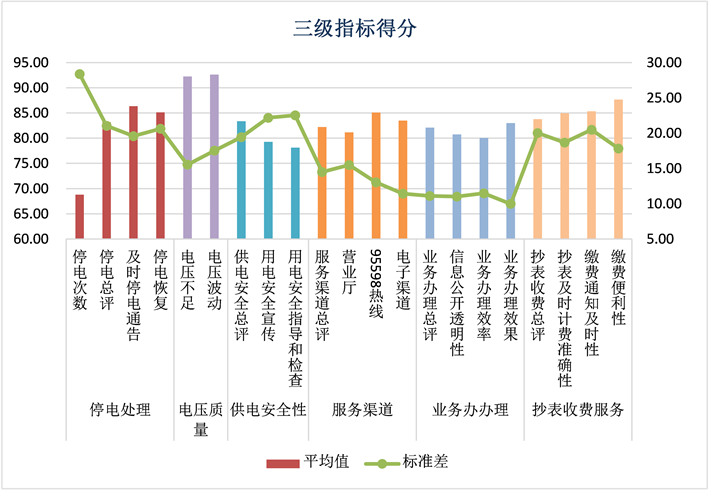Figure 6. Third indicator distribution map

6. 结论

Research on the Improvement Strategy of Grid User Service Satisfaction[J]. 应用数学进展, 2019, 08(02): 242-249. https://doi.org/10.12677/AAM.2019.82028

1. 1. 邹瑶. 电网客户服务满意度研究[D]: [硕士学位论文]. 天津: 天津财经大学, 2017.

2. 2. 曹宇. 基于顾客满意度的XX供电局服务营销策略研究[D]: [硕士学位论文]. 昆明: 云南大学, 2012.

3. 3. 程兰芬. 智能配电网用户满意度评价体系及其在规划项目评判决策中的应用[D]: [硕士学位论文]. 武汉: 华中科技大学, 2013.

4. 4. 蔡飞. 基于顾客满意度佛山供电局服务营销策略研究[D]: [硕士学位论文]. 广州: 华南理工大学, 2011.

5. 5. 常荣. 基于ACSI的电网企业IT呼叫中心客户满意度评价研究[J]. 电力信息与通信技术, 2015, 13(2): 36-42.

6. 6. 闫晓天, 魏宏俊. 电力客户满意度评价体系的建立与应用[J]. 华东电力, 2005, 33(12): 28-29.